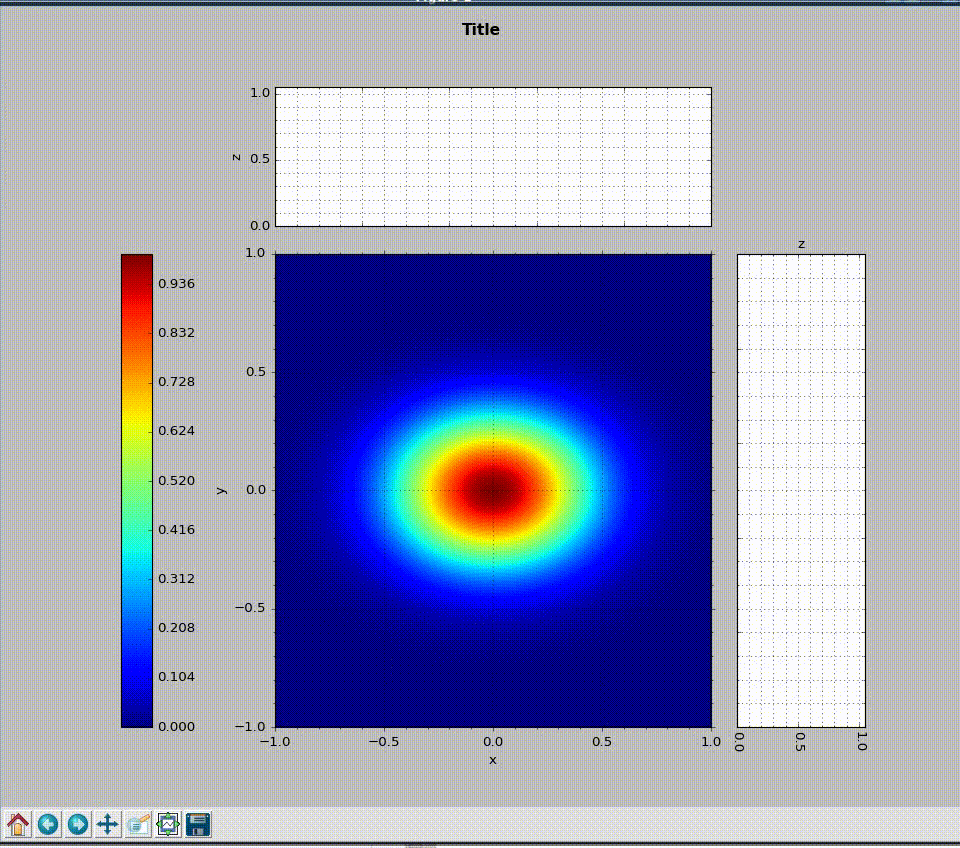# Module Name¶This GitHub repository provides a template to add sphinx / Read The Docs documentation to any python project to generate this Module Docs.

These pages are written using reStructuredText that allows emphasis, strong, literal and many more styles.

You can add a reference [A1], include equations like:

$V(x) = \left(\frac{1-\eta}{\sigma\sqrt{2\pi}}\right) \cdot exp\left({\frac{x^2}{2\sigma^2}}\right) + \eta \cdot \frac{\sigma}{2\pi} \cdot \frac{1}{x^2 + \left(\frac{\sigma}{2}\right)^2}$

or

$I_{white} = \int_{E_{1}}^{E_{2}} I(\theta,E) \cdot F(E)\,dE.$

and tables:

Member Type Example
first ordinal 1st
second ordinal 2nd
third ordinal 3rd

More examples:

$X(e^{j\omega } ) = x(n)e^{ - j\omega n}$

Warning

Warning text.

Note

Note text.## Features¶

• List here
• the module features